# Tristimulus Values and Chromaticity Coordinates

## Tristimulus Values

Fundamentally there are three colors. They are Red (R), Green (G) and Blue (B). Any color that stimulates human eyes is the mixture of R, G, and B in a certain proportion. Let us consider C is the color of an object as the test color. We have taken three sources of R, G, and B color to take an experiment.

The screen is taken to match the color of the test light and the source lights. The upper half of the full screen is taken as Screen 1 and next half is taken as Screen 2. Now the screen 2 is illuminated by the test source C.

We have to match this test source color on the screen 1 by adjusting the R, G and B source colors intensities. The three source colors are so adjusted that we get the main screen without any different color in two halves, i.e. the screen will be with test light color only.

Now we can write as per their intensities thatAs per the figure below the arrangement must be followed by.Here r, g , b are the value of their intensities.
This color matching experiment is taken to get the spectral Tristimulus values of an object color.

As per the above experiment the object color is achieved by adjusting the source color intensity. In the trichromator, it symbolizes the availability of intensities of these three matching stimuli.

If now for arbitrary color that is so selected by adjusting the stimuli R, G and B, the amount of three matching stimuli can be expressed in a new way, that is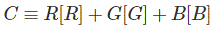Where the symbol ≡ is “read” as matches.

Now the interesting thing is that the monochromatic test stimuli are used to obtain the object color. But practically red color getting mixed with green and blue does not provide exact test object color.

Rather if red is mixed with the test object color then it gives the color same to same as the mixed color of green and blue following perfect intensities. So the color mixture of given amounts of the green and blue matching stimuli will match the mixture of the test and red stimuli. Now the color stimuli equation can be written as: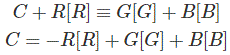This does not mean that red light is negative.
The color matching is additive. 1 unit of power of light with wavelength λ1 [C(λ1)] is matched with the R, G, B primaries, thenand 1 unit of power of light with wavelength λ2 [C(λ2)] is matched with the R,G,B primaries, then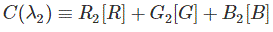then the additive mixture of the two monochromatic lights C(λ1) + C(λ2) will be matched with the additive mixture of the two amounts of the primaries: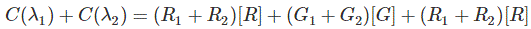The R, G, B Tristimulus values of a stimulus with P(λ) spectral power distribution are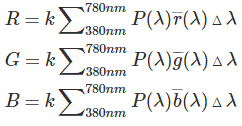Or using integral,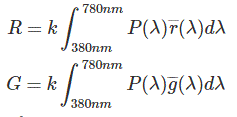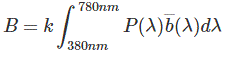The graph of the inverted r(λ), inverted g(λ) and inverted b(λ) color matching functions of the CIE 1931 Standard colorimetric observer is given below.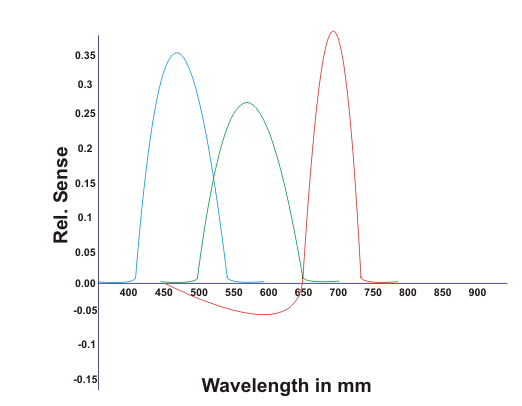## Chromaticity Coordinates

Mainly colors are three types.

1. Source color
2. Object color
3. Derived color

The source color is the color obtained from the source. Whereas object color is the color of an objected when it is illuminated by a perfect white color source.

Again the derived color is the color achieved by mixing of two different colors.
Suppose red (monochromatic) colored source lumen is being projected on the blue (monochromatic) colored object and hence, we get a new appearance of the object color, which is the derived color.

Generally, the functions of wavelength inverted r(λ), inverted g(λ) and inverted b(λ) are represented by inverted x(λ), inverted y(λ) and inverted z (λ).

Now let us suppose inverted x(λ), inverted y(λ) and inverted z(λ) are the functions of the X, Y and Z. Tristimulus values of a color [S(λ)] can be calculated in the same way.Here, S(λ) is the radiometric quantity, and k = 683 lm/W.

The luminance measurement condensed into the Y Tristimulus value. It seemed to be reasonable to transform from the (X, Y, Z) space into another space, Y is one of the coordinates and other two i.e. X and Y is the chromaticity.
The chromaticity coordinates (x, y, z) can be defined as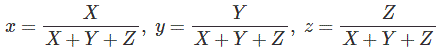where x + y + z = 1. So by using two chromaticity coordinates we can easily describe the chromaticity of stimulus. The chromaticity diagram is given below.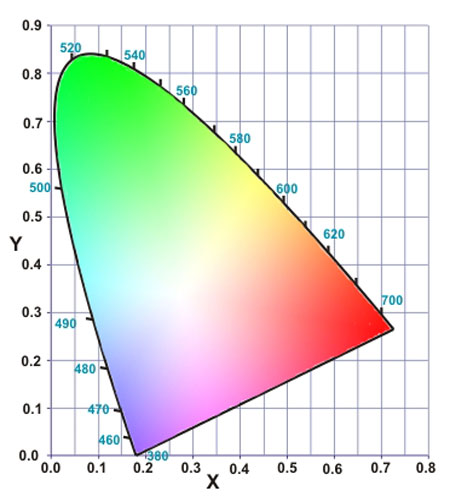The chromaticity point of two additive mixed colors is located on the line joining the chromaticity points of the two constituent colors in this chromaticity diagram.

The mixture of red and blue gives the purple color. In this diagram the locus covered by R, G and B give the continuous wavelength, whereas the side of purple does not give the continuous wavelength, rather it is discontinuous.

Chromaticity of the Additive Mixture of Two Stimuli:
If aR amount of red is mixed with aG amount of green then the Tristimulus values of the additive mixture color will be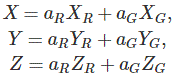So the corresponding chromaticity coordinates are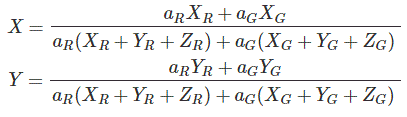The amount of luminance of red stimulus is represented as aR YR, the amount of luminance of green stimulus is represented as aG XG.
This is also called the center of the gravity of law of the color mixture.

Want To Learn Faster? 🎓
Get electrical articles delivered to your inbox every week.
No credit card required—it’s 100% free.